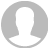﻿ MATLAB之函数与预算_Moore8摩尔吧## MATLAB之函数与预算

29 人已报名

• 课程概述
• 课程目录
• 学员评价
• 课程问答

Matlab函数与预算

1 MATLAB7.0的特殊矩阵与数组

2 MATLAB7.0的多项式

3 MATLAB7.0的关系运算与逻辑运算

MATLAB中，求解矩阵A的特征值和特征向量的函数是eigA